Effects of rough surface scattering on terahertz radar imaging
OU Zhan, ZHENG Xiaoping, GENG Hua
Department of Automation, Tsinghua University, Beijing 100084, China
Abstract: Traditional millimeter wave security imaging radar ignores the effects of scattering due to the point target assumption. However, for frequencies in the terahertz band, the target surface should be modeled as a rough surface and the effects of scattering must be considered. In this paper, a small perturbation method (SPM) is used to get the backscattering coefficient of a rough surface. Then, the imaging results for the traditional security radar imaging model are simulated for various roughnesses. The effects of key scattering parameters including the roughness and frequency are then analyzed by comparisons with a Lambert surface and an ideal specular surface. The results show that a greater backscattering persistence angle improves the imaging quality with terahertz security radar imaging. The results also show that the angular width and shape of the backscattering characteristic curve both affect the imaging resolution and peak side lobe ratio. Finally, increasing the electromagnetic wave frequency facilitates rough surface imaging.
Key words: terahertz     radar imaging     rough surface scattering     small perturbation method

1 基于微扰法的散射成像模型

1.1 微扰法

 ${\sigma _{pq}} = 8{k^4}{\delta ^2}{\cos ^4}{\theta _{\rm{i}}}{\left| {{\alpha _{pq}}} \right|^2}W\left( {2k\sin {\theta _{\rm{i}}}} \right).$ (1)

 ${\alpha _{{\rm{HH}}}} = \frac{{1 - {\varepsilon _{\rm{r}}}}}{{{{\left[ {\cos {\theta _{\rm{i}}} + {{\left( {{\varepsilon _{\rm{r}}} - \sin {\theta _{\rm{i}}}} \right)}^{1/2}}} \right]}^2}}}.$ (2)

 $W\left( {2{k_1}\sin {\theta _{\rm{i}}}} \right) = \frac{1}{2}{l^2}\exp \left[ { - {{\left( {kl\sin {\theta _{\rm{i}}}} \right)}^2}} \right].$ (3)

1.2 后向散射模型

 表面类型 散射系数 Lambert表面(点目标) σ=const (0 < θi < π/2) 镜反射表面 σ=const (0 < θi < θscatter) 粗糙表面 SPM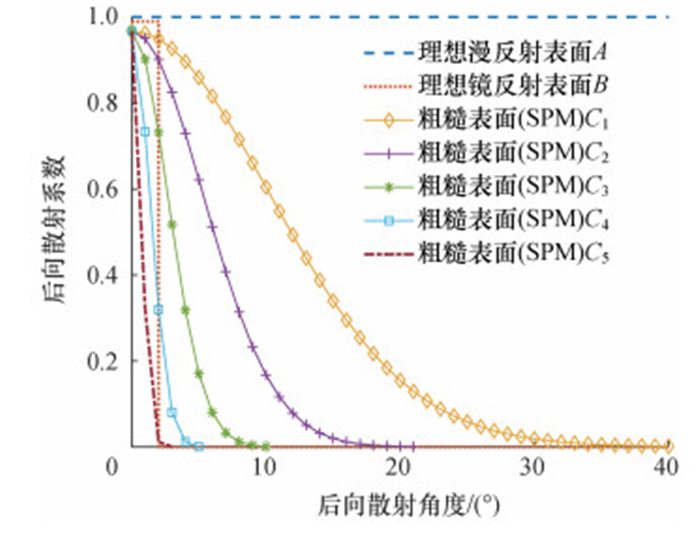图 1 不同表面的后向散射系数角分布

1.3 成像模型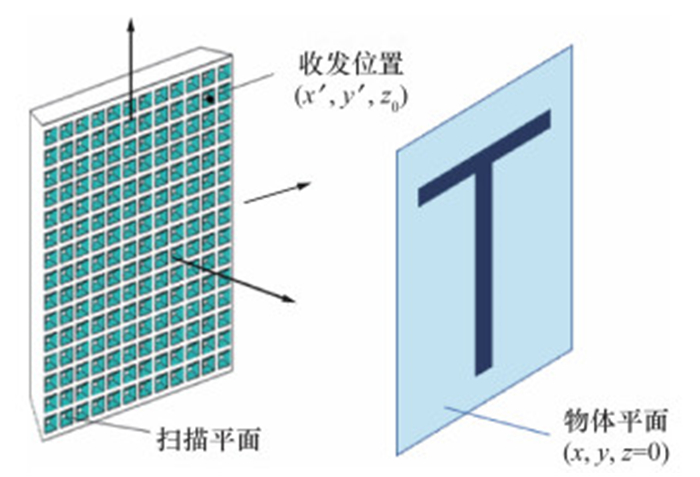图 2 经典的安检雷达成像场景

 $s\left( {{x^\prime }, {y^\prime }} \right) = \int\int {(x, y)\frac{1}{{{r^2}}}\exp ( - {\rm{j}}2kr){\rm{d}}x{\rm{d}}y.}$ (4)

 $s\left( {{x^\prime }, {y^\prime }} \right) = \int\int {\left( {x, y, {\theta _{\rm{i}}}} \right)\frac{1}{{{r^2}}}\exp ( - {\rm{j}}2kr){\rm{d}}x{\rm{d}}y.}$ (5)

 $\begin{array}{*{20}{c}} {\sigma \left( {x,y,{\theta _{\rm{i}}}} \right) = \sigma (x,y) = }\\ {{\rm{FT}}_{2{\rm{D}}}^{ - 1}\left[ {{\rm{F}}{{\rm{T}}_{2{\rm{D}}}}\left[ {s\left( {{x^\prime },{y^\prime }} \right)} \right]\exp \left( { - {\rm{j}}k_z^\prime {z_0}} \right)} \right].} \end{array}$ (6)

2 仿真分析

2.1 PSF图 3 不同表面的PSF传统的成像分辨率计算公式为

 $\delta \approx \frac{\lambda }{{4\sin {\theta _{\rm{b}}}}}.$ (7)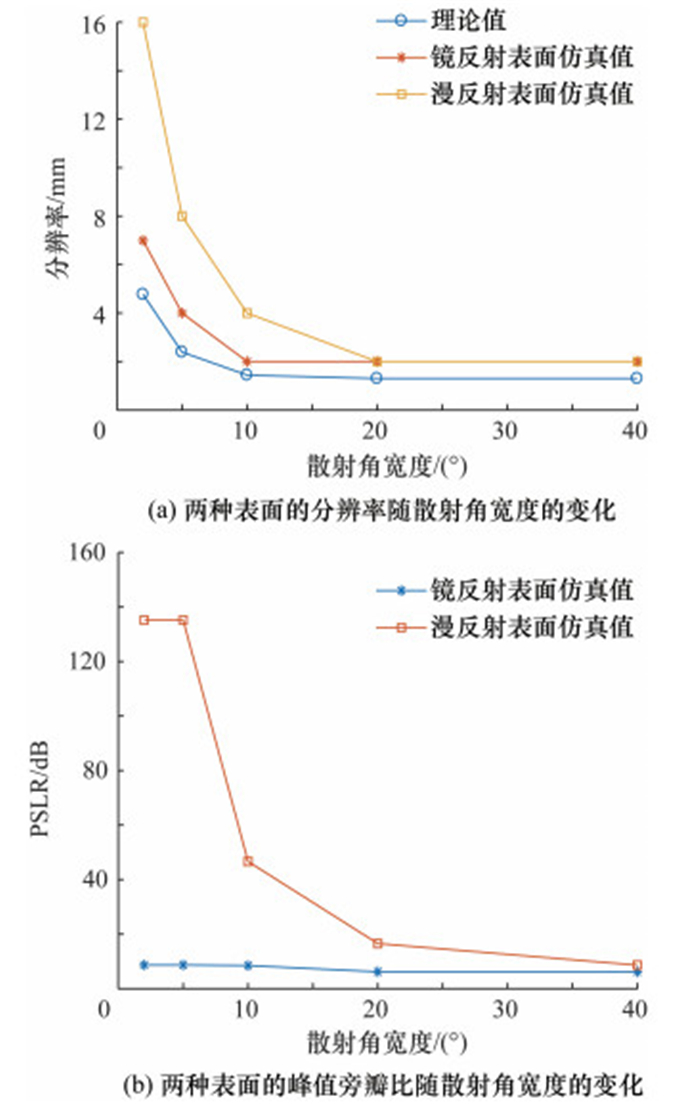图 4 镜反射表面与漫反射表面的分辨率与峰值旁瓣比

2.2 单目标成像图 5 “T”字形目标示意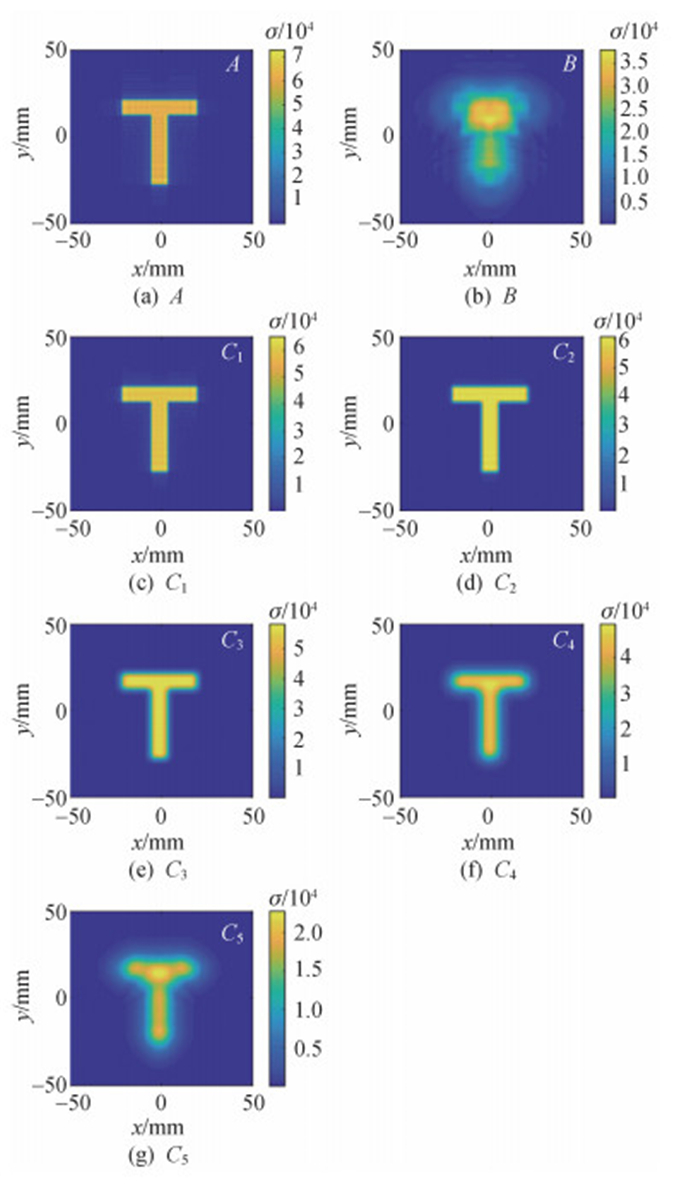图 6 不同表面的“T”字形目标成像结果

2.3 多目标成像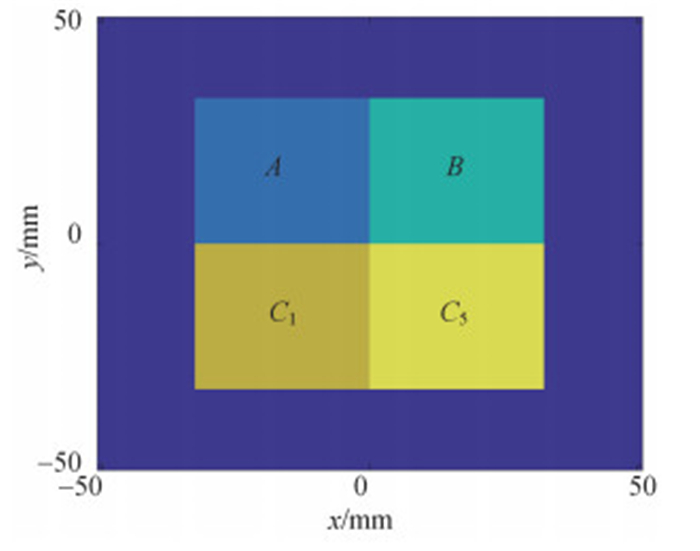图 7 多目标示意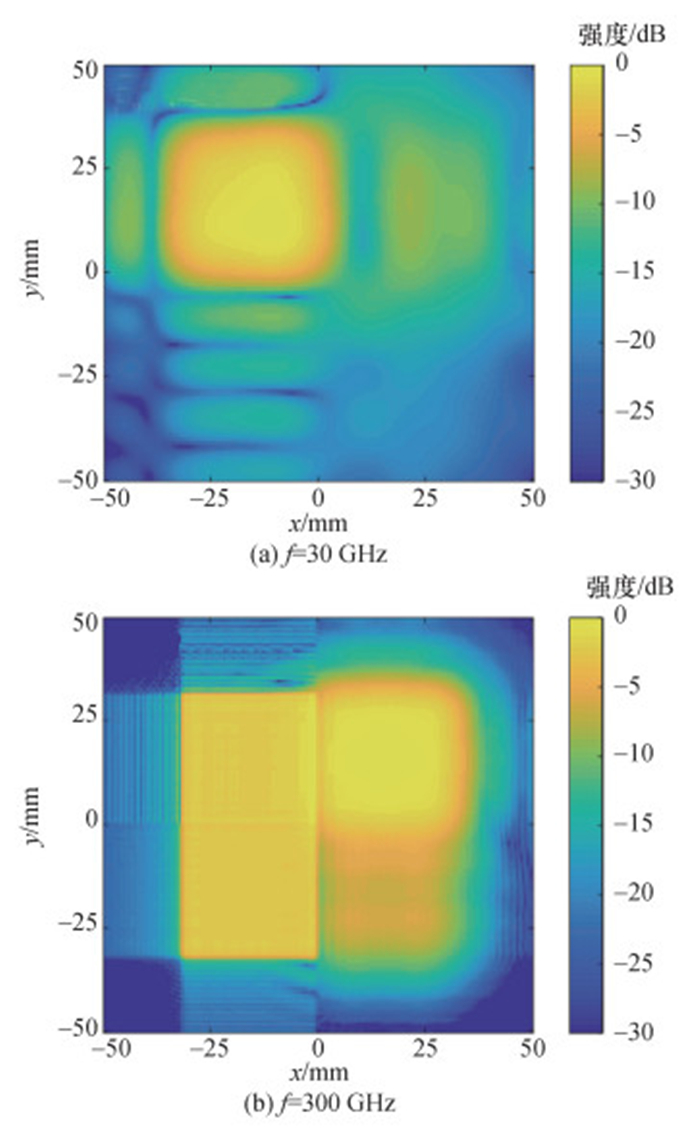图 8 多目标成像结果

 太赫兹波频率/GHz 强度/dB A B C1 C5 30 -2.1 -10.6 -14.5 -15.6 300 -1.8 -2.1 -2.2 -8.6

3 结论

1) 在忽略天线方向图和相干斑噪声的情况下，材料表面粗糙度越大，后向散射角越宽，成像分辨率越高，成像质量越好。

2) 散射特性曲线的角宽度和曲线形状共同影响着成像系统分辨率和峰值旁瓣比。相同散射角宽度时，漫反射表面比镜反射表面分辨率更低，但峰值旁瓣比更高。

3) 提高电磁波频率有利于粗糙表面成像。

  DHILLON S S, VITIELLO M S, LINFIELD E H, et al. The 2017 terahertz science and technology roadmap[J]. Journal of Physics D:Applied Physics, 2017, 50(4): 043001. DOI:10.1088/1361-6463/50/4/043001  SHEEN D M, MCMAKIN D L, HALL T E. Three-dimensional millimeter-wave imaging for concealed weapon detection[J]. IEEE Transactions on Microwave Theory and Techniques, 2002, 49(9): 1581-1592.  SHEEN D, MCMAKIN D, HALL T. Near-field three-dimensional radar imaging techniques and applications[J]. Applied Optics, 2010, 49(19): E83-E93. DOI:10.1364/AO.49.000E83  SHEEN D M, FERNANDES J L, TEDESCHI J R, et al. Wide-bandwidth, wide-beamwidth, high-resolution, millimeter- wave imaging for concealed weapon detection[C]//Proceedings of SPIE 8715, Passive and Active Millimeter-Wave Imaging XVI. Baltimore, USA: SPIE, 2013: 871509.  COOPER K B, DENGLER R J, LLOMBART N, et al. THz imaging radar for standoff personnel screening[J]. IEEE Transactions on Terahertz Science and Technology, 2011, 1(1): 169-182. DOI:10.1109/TTHZ.2011.2159556  ALEXANDER N E, ALDERMAN B, ALLONA F, et al. TeraSCREEN: Multi-frequency multi-mode terahertz screening for border checks[C]//Proceedings of SPIE 9078, Passive and Active Millimeter-Wave Imaging XVⅡ. Baltimore, USA: SPIE, 2014: 907802.  GU S M, LI C, GAO X, et al. Terahertz aperture synthesized imaging with fan-beam scanning for personnel screening[J]. IEEE Transactions on Microwave Theory and Techniques, 2012, 60(12): 3877-3885. DOI:10.1109/TMTT.2012.2221738  崔振茂, 高敬坤, 陆彬, 等. 340 GHz稀疏MIMO阵列实时3-D成像系统[J]. 红外与毫米波学报, 2017, 36(1): 102-106. CUI Z M, GAO J K, LU B, et al. Real time 3D imaging system based on sparse MIMO array at 340GHz[J]. Journal of Infrared and Millimeter Waves, 2017, 36(1): 102-106. (in Chinese)  XU F, WANG P. Analytical modeling of rough surface SAR images under small perturbation approximation[J]. IEEE Transactions on Geoscience and Remote Sensing, 2017, 55(7): 3694-3707. DOI:10.1109/TGRS.2017.2677525  GROSSMAN E N, POPOVIC N, CHAMBERLIN R A, et al. Submillimeter wavelength scattering from random rough surfaces[J]. IEEE Transactions on Terahertz Science and Technology, 2017, 7(5): 546-562. DOI:10.1109/TTHZ.2017.2725481  DIGIOVANNI D A, GATESMAN A J, GOYETTE T M, et al. Surface and volumetric backscattering between 100 GHz and 1.6 THz[C]//Proceedings of SPIE 9078, Passive and Active Millimeter-Wave Imaging XVⅡ. Baltimore, USA: SPIE, 2014: 90780A.  WEI J C, CHEN H, QIN X, et al. Surface and volumetric scattering by rough dielectric boundary at terahertz frequencies[J]. IEEE Transactions on Antennas and Propagation, 2017, 65(6): 3154-3161. DOI:10.1109/TAP.2017.2690307  JANSEN C, PRIEBE S, MOLLER C, et al. Diffuse scattering from rough surfaces in THz communication channels[J]. IEEE Transactions on Terahertz Science and Technology, 2011, 1(2): 462-472. DOI:10.1109/TTHZ.2011.2153610  SUNDBERG G, ZURK L M, SCHECKLMAN S, et al. Modeling rough-surface and granular scattering at terahertz frequencies using the finite-difference time-domain method[J]. IEEE Transactions on Geoscience and Remote Sensing, 2010, 48(10): 3709-3719. DOI:10.1109/TGRS.2010.2048717  PÄTZOLD M, KAHL M, KLINKERT T, et al. Simulation and data-processing framework for hybrid synthetic aperture THz systems including THz-scattering[J]. IEEE Transactions on Terahertz Science and Technology, 2013, 3(5): 625-634. DOI:10.1109/TTHZ.2013.2274698  APPLEBY R, PETERSSON H, FERGUSON S. Concealed object stand-off real-time imaging for security: CONSORTIS[C]//Proceedings of SPIE 9462, Passive and Active Millimeter-Wave Imaging XVⅢ. Baltimore, USA: SPIE, 2015: 946204.  ORTIZ-JIMÉNEZ G, GARCÍA-RIAL F, AU'G BEDA-MEDINA L A, et al. Simulation framework for a 3-D high-resolution imaging radar at 300GHz with a scattering model based on rendering techniques[J]. IEEE Transactions on Terahertz Science and Technology, 2017, 7(4): 404-414. DOI:10.1109/TTHZ.2017.2702590  郭立新, 王蕊, 吴振森. 随机粗糙面散射的基本理论与方法[M]. 北京: 科学出版社, 2010. GUO L X, WANG R, WU Z S. Basic theory and method of random rough surface scattering[M]. Beijing: Science Press, 2010. (in Chinese)  VARSHNEY K R, ÇETIN M, FISHER J W, et al. Sparse representation in structured dictionaries with application to synthetic aperture radar[J]. IEEE Transactions on Signal Processing, 2008, 56(8): 3548-3561. DOI:10.1109/TSP.2008.919392